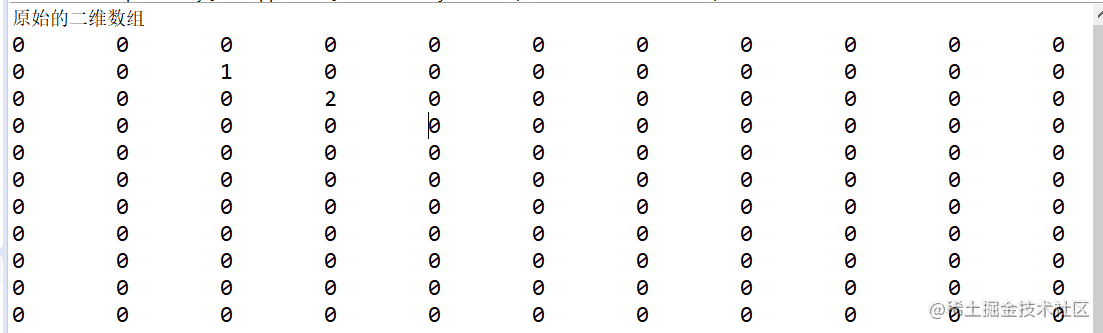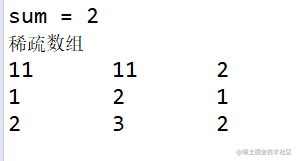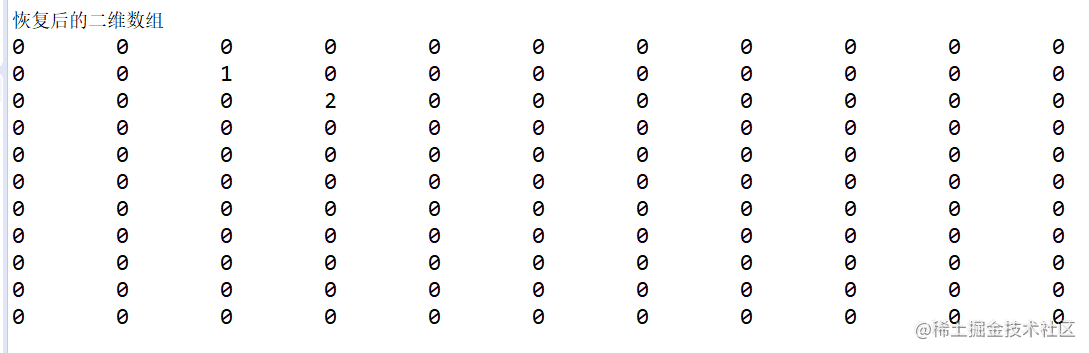# 数据结构----稀疏数组

## 代码示例

`使用代码模拟棋盘（二维数组）`

``````public static void main(String[] args) {
//棋盘上 0 表示 没有棋子 ，1 表示黑子 2 表示红子
//创建一个原始的二维数组，当作棋盘
int chessArr1[][] = new int;
//给棋盘上一些值
chessArr1 = 1;
chessArr1 = 2;
//打印棋盘
//便利数组每一行的值赋给 row
System.out.println("原始的二维数组");
for(int[] row : chessArr1) {
//便利 row 的值 赋值给 data
for(int data : row) {
//格式化打印棋盘上的数据
System.out.printf("%d\t",data);
}
//换行
System.out.println();
}
}

#### 上述代码模拟实现了五子棋中棋盘落子的情况，其中 1 表示 黑子，2 表示 红子 ，0 表示 未下子

`执行结果``将二维数组转换为稀疏数组`

``````public static void main(String[] args) {

//将二维数组转换为稀疏数组
//1. 遍历 二维数组 得到非0 数据的个数
//非0 个数
int sum = 0;
//遍历二维数组的行数
for (int i = 0; i < chessArr1.length; i++) {
//遍历二维数组的列数
for (int j = 0; j < chessArr1.length; j++) {
//值不为0 将他的个数记录下来
if(chessArr1[i][j] !=0 ) {

sum ++ ;
}
}
}
System.out.println("sum = "+sum);
//创建稀疏数组
//稀疏数组 的 行为有效数据中个数+1 固定时3 列
int sparseArr[][] = new int[sum+1];
//稀疏数组 第一行第一列的数据
sparseArr = chessArr1.length;
//稀疏数组 第一行第2列的数据
sparseArr = chessArr1.length;
//稀疏数组 第一行第3列的数据
sparseArr = sum;
//遍历二维数组将 非0 数据放入稀疏数组
//计数器 ，用来计算 当前数第几行
int count = 0 ;
for (int i = 0; i < chessArr1.length; i++) {
for (int j = 0; j < chessArr1.length; j++) {
//当二维数组中数据不为0 时 放到稀疏数组中
if(chessArr1[i][j]!=0) {
count ++;
//稀疏数组从第二行开始 每列的数值
sparseArr[count] = i;
sparseArr[count] = j;
sparseArr[count] = chessArr1[i][j];
}
}
}
//输出稀疏数组
System.out.println("稀疏数组");
for (int i = 0; i < sparseArr.length; i++) {
System.out.printf("%d\t%d\t%d\t\n",sparseArr[i],sparseArr[i],sparseArr[i]);
}
System.out.println("");

`执行结果```````其中因为稀疏数组的列是固定的3 列 ，而且 第一行第一例的数据是二维数组的总行数，第一行第二列的数据是 二维数组的总列数，第一行 第三列的数据是二维数组中有效数据（不为0）的总个数

`将稀疏数组转换为二维数组`

``````public static void main(String[] args) {
//将稀疏数组 转为 二维数组
//1. 稀疏数组的第一行 第一列和第二列 存放的是 二维数组的行列
int chessArr2[][] = new int[sparseArr][sparseArr];
//2. 从第二行开始将 稀疏数组中的数据赋值给 二维数组 因为都是有效数据 ，不用判断为0
for (int i = 1; i < sparseArr.length; i++) {
chessArr2[sparseArr[i]][sparseArr[i]] = sparseArr[i];
}
System.out.println("恢复后的二维数组");
for(int[] row : chessArr2) {
//便利 row 的值 赋值给 data
for(int data : row) {
//格式化打印棋盘上的数据
System.out.printf("%d\t",data);
}
//换行
System.out.println();
}
}

``````在转换回去时我们从稀疏数组的第一行第一列，第一行第二列 获取需要创建的二维数组的大小

`执行结果`